UPDATE: This blog post is still good for learning DAX principles, but a better description of many-to-many patterns is available on The Many-to-Many Revolution whitepaper that is available here: http://www.sqlbi.com/articles/many2many/ – please download it and use the pattern described there to write faster DAX formulas!

PowerPivot doesn’t have the capability of really understand a many-to-many (M2M) relationship between two tables. In a relational world, a many-to-many relationship is materialized using a bridge table that split this relationship in two separate one-to-many relationships between the two original tables and the bridge table. Apparently, we can do the same in PowerPivot, but the behavior is not the expected one. Some workaround is possible using DAX, but there are some undesirable side effects if we only use calculated column. As we will see, it is necessary to use calculated measures to get the best results.

Consider two tables, Customers and Accounts.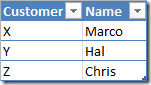Customers Table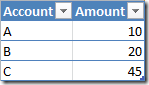Accounts Table

The many-to-many relationship between Customers and Accounts is expressed by the following Cross table.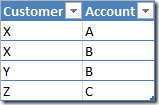Cross Table

In PowerPivot we define the same three tables (Customers, Accounts and Cross) and then we define these relationships: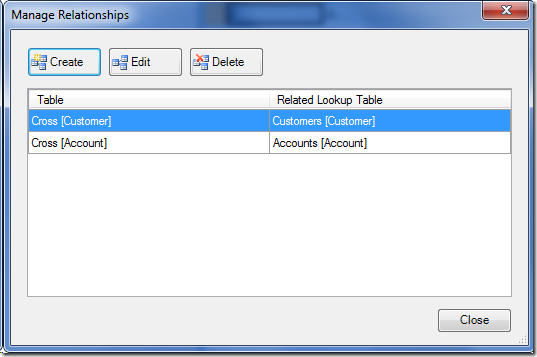As we will see, the best result is obtained using calculated measures. However, it is interesting to look at the results that can be obtained defining calculated columns, just to understand how PowerPivot works under the hood. You can skip the following section going directly to “Defining Calculated Measures” if you just want to look at the best solution. But if you want to understand how it works, it is better to start from the beginning, looking at the calculated columns before.

## Defining Calculated Columns

At this point, we can try the PivotTable using the Amount measure next each Customer, but the result will sum up all the accounts without filtering only the accounts belonging to the Customer in the same resulting row.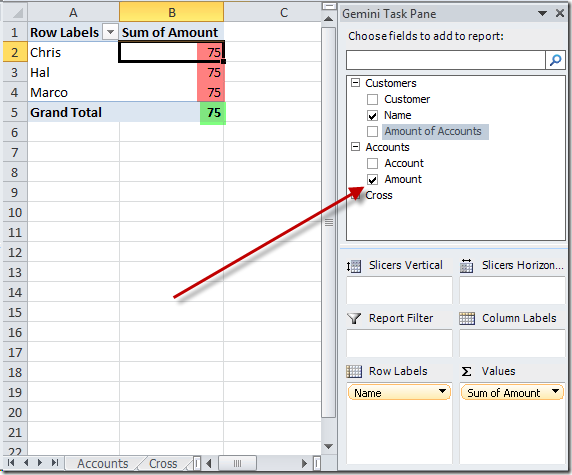The value of Grand Total is right, because it represents the sum or all the accounts – but this is also the same value for each customer and this is not right.

We can solve this by adding a DAX expression into a new column in the Customers table. We will call this column Amount of Accounts.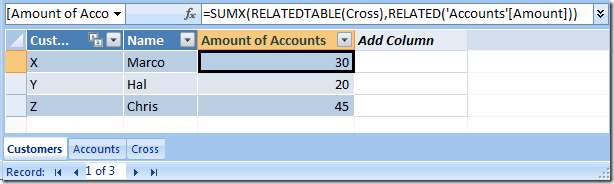The DAX expression will sum (SUMX) all the value of the column Amounts in the Accounts table (‘Accounts’[Amount]) that are related (RELATED(‘Accounts’[Amount])) to the rows in the Cross table considered for each customer (RELATEDTABLE(Cross)). The final result is the desired value for each single customer.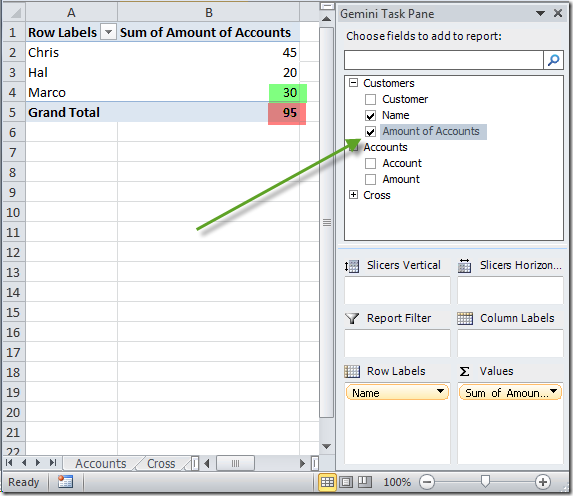As you can see, Marco has a total of 30, which is the sum of the accounts A (10) and B (20). However, the Grand Total is now 95, which is right if you sum the amount of each customer, but it is wrong if you consider that the same account (B) is considered for both customers Marco and Hal. In other words, if these were bank accounts, looking at this number we would be saying that the bank has more deposits than real.

Moreover, if you want to analyze which are the accounts accessible by each customer, dicing Customers and Accounts doesn’t filter out the accounts that don’t belong to the analyzed Customer. In the following screenshot you can see that all the accounts are present with the total amount of the accounts belonging to analyzed Customer.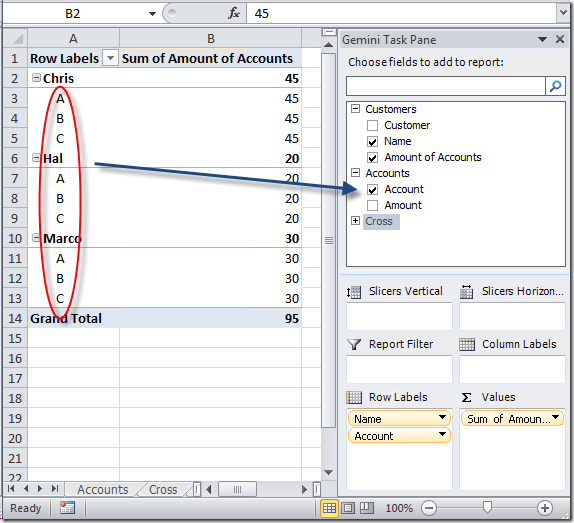We can obtain a better result with a model that is less intuitive from the point of view of the final user. We can create a RelatedAmount calculated column in the Cross table using the DAX expression RELATED(Accounts[Amount]).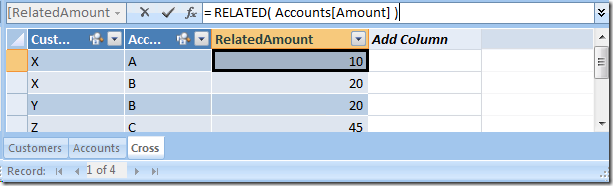In this way, we will use the implicit selection on Cross table made by the relationships we created before in the PowerPivot model, and the final result will also consider the relationship existing between the Cross and the Accounts tables to filter out Accounts which are not related to the selected Customer. Moreover, the value for each account will also be right.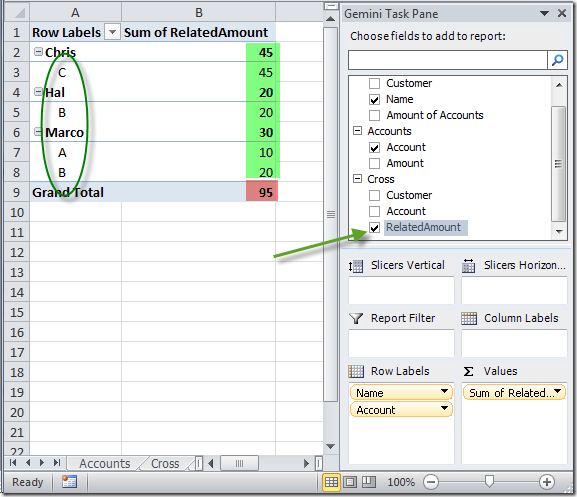However, the Grand Total is still higher than the real amount of all accounts.
This work-around still is not ideal, even because the more intuitive place for the end user for having the amount for all the customers is the Customers table, and not the Cross table which should be invisible to the end user (it is a service table and shouldn’t have meaningful information for the data analyst).

## Defining Calculated Measures

The best solution is defining a calculated measure in the Customers table (right click the Customers node in the PowerPivot Task Pane):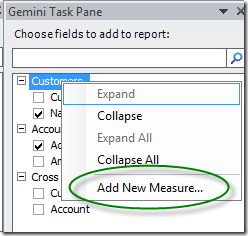We define a formula that sums the amount of all the accounts that have at least a line in the Cross table which is related to the context of the calculation in the PivotTable (we will analyze the formula in details later).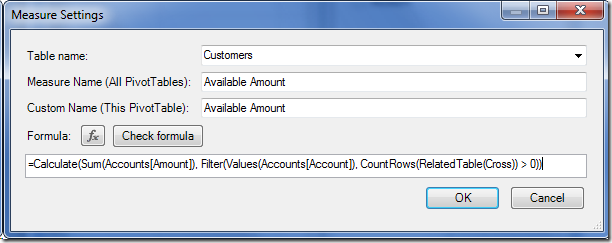The final result in our PivotTable is exactly what we wanted: right results for accounts and customers, exclusion of the accounts which are not owned by a customer, right total for all customers. Moreover, the measure we defined (Available Amount) can be defined wherever we want, even in the Customers table (like we did).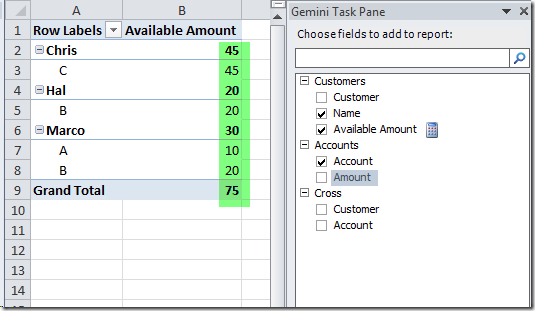Now, the problem is only that the Amount value in the Accounts table might be misbelieving (look at the first example in the previous “Defining Calculated Columns” section). However, we can hide a column (even if we cannot hide a calculated measure, like the “Available Amount” we just created using the PivotTable).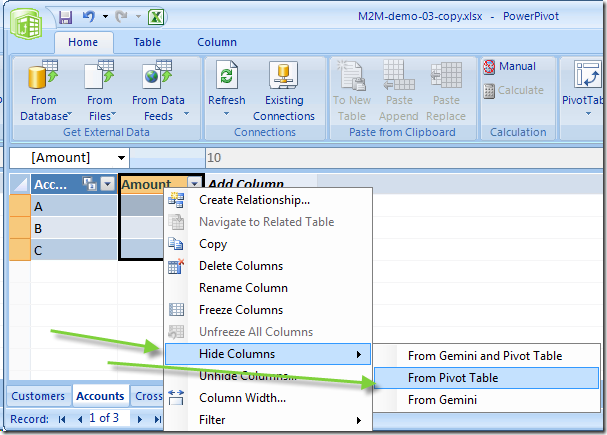At this point, we can also move the calculated measure under the Accounts table, which seems the more intuitive place to look for, especially if there aren’t other measures with similar names.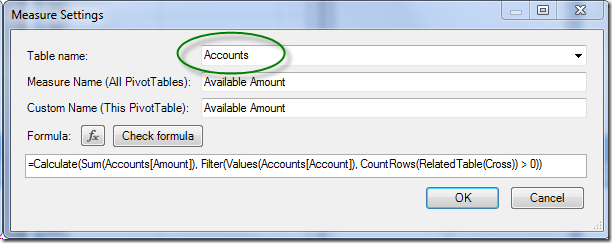In this way, we have the cleanest presentation for the PivotTable user.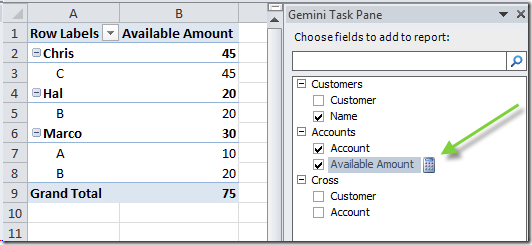### Calculated Measures Under The Hood

To understand how we created the Available Amount calculated measure, we have to split it into smaller terms (I thanks Marius Dumitru for his help in clarifying me these steps). In the following steps, we will keep the original “Accounts[Amount]” column hidden from the PivotTable.

First of all, we define these two calculated measures:

• Accounts[Amt] = Sum( [Amount] )
• Cross[x] = CountRows(Cross)

Apparently, these two formulas calculate the sum of all the rows in the corresponding tables (Accounts and Cross). But, remember, we are defining calculated measures and these formulas are executed in a particular execution context for each cell of the resulting PivotTable. Look at the following picture.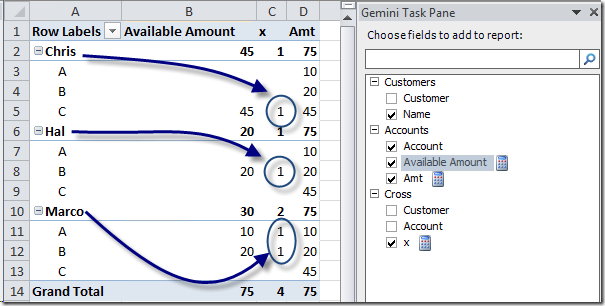The x calculation has been filtered by the selection of Customers and Accounts: this is regular, because the Cross table is like a fact table, which is filtered by the selections made on all of the dimensions. However, the Amt calculation is filtered only by Account selection and is not affected by the Customer in the context. In fact, there are not direct relationships between Customers and Accounts tables. To get the right Amount value, we need to filter the Amt sum also by Accounts that are owned by selected Customers, and to do this, we can filter only the rows in the Account table that have at least one corresponding row in the Cross table for the current Account and Customer in the execution context. For this reason we use the FILTER function to get the accounts for the current selection of customers. These accounts will have at least one row in the Cross table, which is related to the Customer table, and this predicate will filter all the accounts we have to sum to get the desired result. The following expression implements this logic, returning the set of accounts to be summed for the current context.

( Filter(Values(Accounts[Account]), [x] > 0) )

At this point, it is sufficient to reiterate this process for each cell that have to be calculated. The same formula can be written with a shorter version that eliminates the CALCULATE syntax.

Calculate( [Amt], ( Filter(Values(Accounts[Account]), [x] > 0) ) )

[Amt] ( Filter(Values(Accounts[Account]), [x] > 0) )

Please note that the calculated measures [Amt] and [x] doesn’t need to be preceded by the name of the table – other than calculated columns, the name of the owning tables has only two purposes for calculated measures:

• Providing a “default table” that will be used when you write simple aggregation functions without specifying a table: writing Sum([C]) in a measure will get expanded to Sum(DT[C]) (or SumX(DT, [C])), where DT is the table that the measure is defined on.
• Defining where (under which table) the UI should the measures in the FieldList (like DisplayFolder does in MDX).

Thus, starting from this formula:

Calculate( [Amt], ( Filter(Values(Accounts[Account]), [x] > 0) ) )

We can obtain our [Available Amount] calculated measure by simply replacing [Amt] and [x] terms (we use the Accounts[Amount] explicit term so that the formula can be defined under any table of the model):

Calculate( Sum(Accounts[Amount]), ( Filter(Values(Accounts[Account]), CountRows(RelatedTable(Cross)) > 0) ) )

The following ones are alternative ways of writing the same calculation (please note we are using an additive measure like Amount – in case of non-additive measures, the SumX alternatives don’t work well).

Calculate(Sum([Amount]), Filter(Values(Accounts[Account]), Calculate(CountRows(Cross)) > 0))

SumX(Filter(Values(Accounts[Account]), CountRows(RelatedTable(Cross)) > 0), SumX(RelatedTable(Accounts), [Amount]))

SumX(Filter(Values(Accounts[Account]), CountRows(RelatedTable(Cross)) > 0), Calculate(Sum([Amount])))

SumX(Filter(Values(Accounts[Account]), CountRows(RelatedTable(Cross)) > 0), [Amt])

## Final considerations

When you look at PowerPivot, you might think that calculated columns and calculated measures are pretty similar, just because they share the same expression language (DAX). However, they are really different and provide complementary tools to implement more advanced calculations. I don’t like the fact that calculated measures belong to the PowerPivot model but can be defined only using a PivotTable. I would prefer having also a dedicated tool into the PowerPivot window to access calculated measures definitions.

Calculated measures are flexible enough to make us implement a many-to-many relationship calculation. This is a very important feature to handle more complex models presenting data in an intuitive way. I’m just at the beginning of the exploration of these capabilities and I still hadn’t time to analyze performance and scalability of such a calculations. In the future, I’d like to compare features and performance with equivalent “traditional” SSAS models.

SUMXReturns the sum of an expression evaluated for each row in a table.

`SUMX ( <Table>, <Expression> )`

RELATEDTABLEContext transition

Returns the related tables filtered so that it only includes the related rows.

`RELATEDTABLE ( <Table> )`

FILTERReturns a table that has been filtered.

`FILTER ( <Table>, <FilterExpression> )`

CALCULATEContext transition

Evaluates an expression in a context modified by filters.

`CALCULATE ( <Expression> [, <Filter> [, <Filter> [, … ] ] ] )`